Search by Topic

Resources tagged with Recording mathematics similar to Round the Dice Decimals 1:

Filter by: Content type:
Age range:
Challenge level:

There are 18 results

Broad Topics > Using, Applying and Reasoning about Mathematics > Recording mathematicsRound the Dice Decimals 1

Age 7 to 11 Challenge Level:

Use two dice to generate two numbers with one decimal place. What happens when you round these numbers to the nearest whole number?Round the Dice Decimals 2

Age 7 to 11 Challenge Level:

What happens when you round these numbers to the nearest whole number?Round the Three Dice

Age 7 to 11 Challenge Level:

What happens when you round these three-digit numbers to the nearest 100?How Do You See It?

Age 5 to 11 Challenge Level:

Here are some short problems for you to try. Talk to your friends about how you work them out.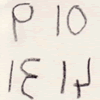Children's Mathematical Writing

Age 5 to 11

Bernard Bagnall discusses the importance of valuing young children's mathematical representations in this article for teachers.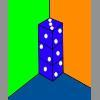Dice in a Corner

Age 7 to 11 Challenge Level:

How could you arrange at least two dice in a stack so that the total of the visible spots is 18?Multiply Multiples 3

Age 7 to 11 Challenge Level:

Have a go at balancing this equation. Can you find different ways of doing it?Multiply Multiples 1

Age 7 to 11 Challenge Level:

Can you complete this calculation by filling in the missing numbers? In how many different ways can you do it?Multiply Multiples 2

Age 7 to 11 Challenge Level:

Can you work out some different ways to balance this equation?Round the Four Dice

Age 7 to 11 Challenge Level:

This activity involves rounding four-digit numbers to the nearest thousand.Age 5 to 11 Challenge Level:

How could you put these three beads into bags? How many different ways can you do it? How could you record what you've done?Junior Frogs

Age 5 to 11 Challenge Level:

Have a go at this well-known challenge. Can you swap the frogs and toads in as few slides and jumps as possible?Plants

Age 5 to 11 Challenge Level:

Three children are going to buy some plants for their birthdays. They will plant them within circular paths. How could they do this?More Children and Plants

Age 7 to 14 Challenge Level:

This challenge extends the Plants investigation so now four or more children are involved.More Plant Spaces

Age 7 to 14 Challenge Level:

This challenging activity involves finding different ways to distribute fifteen items among four sets, when the sets must include three, four, five and six items.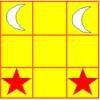Knight's Swap

Age 7 to 11 Challenge Level:

Swap the stars with the moons, using only knights' moves (as on a chess board). What is the smallest number of moves possible?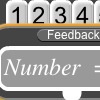Exploring Number Patterns You Make

Age 7 to 11 Challenge Level:

Explore Alex's number plumber. What questions would you like to ask? What do you think is happening to the numbers?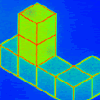Pupils' Recording or Pupils Recording

Age 5 to 14

This article, written for teachers, looks at the different kinds of recordings encountered in Primary Mathematics lessons and the importance of not jumping to conclusions!